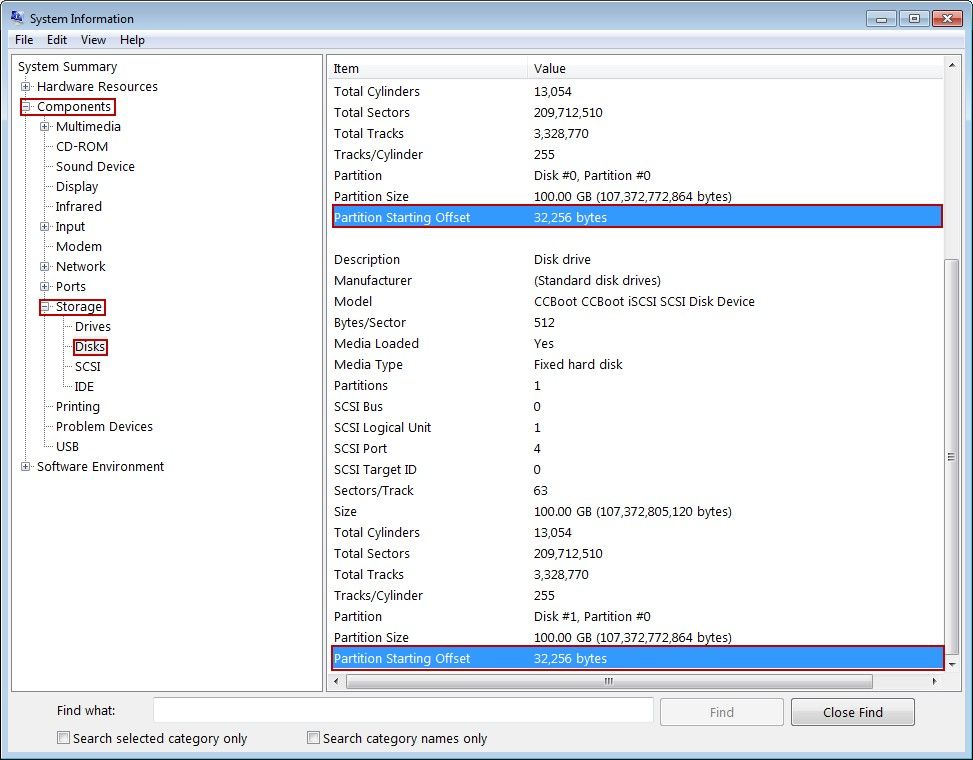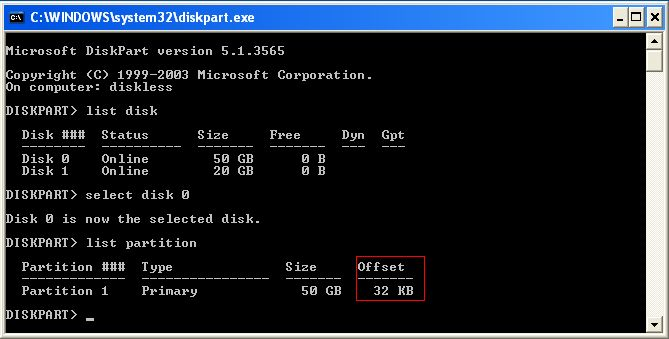Wiki Home Disk Manager How to Check Whether the HDD Is 4K Aligned

How to Check Whether the HDD Is 4K Aligned

2014/07/17 18:11

In fact, whether the HDD is 4K aligned does not matter much. But you can check it according to the following steps.

1) In Windows Vista/7 operating system, click the "Start" button, in the "Run" edit box, type "msinfo32", and then press the "Enter" key.

2) It will pop up the "System Information" form. In the left side of the form, on the directory tree, click the "Components" node, expand the directory tree. Click the "Storage" node, expand the directory tree, and then select the "Disks" node (Figure 1-1).Figure 1-1

3) Check the "Partition Starting Offset" value. If the value cannot be divided by 4096, then the HDD is not 4K aligned. If the value can be divided by 4096, then the HDD is 4K aligned, and does not need the corresponding partition alignment tool for further operations.

4) From Figure 1-1, we can know that these two partitions' "Partition Starting Offset" value is 32,256, which can not be divided by 4096. So, these two HDDs are not 4K aligned.

Note: This problem will not happen if using the Win7 built-in HDD tool to format the disks.

If it's XP system, you can check the 4K alignment by the following command.

1) Run -> diskpart
2) list disk
3) select disk 0
4) list partition

You will see the Offset is 32K. That means disk0 partition 1 is 4K alignment.